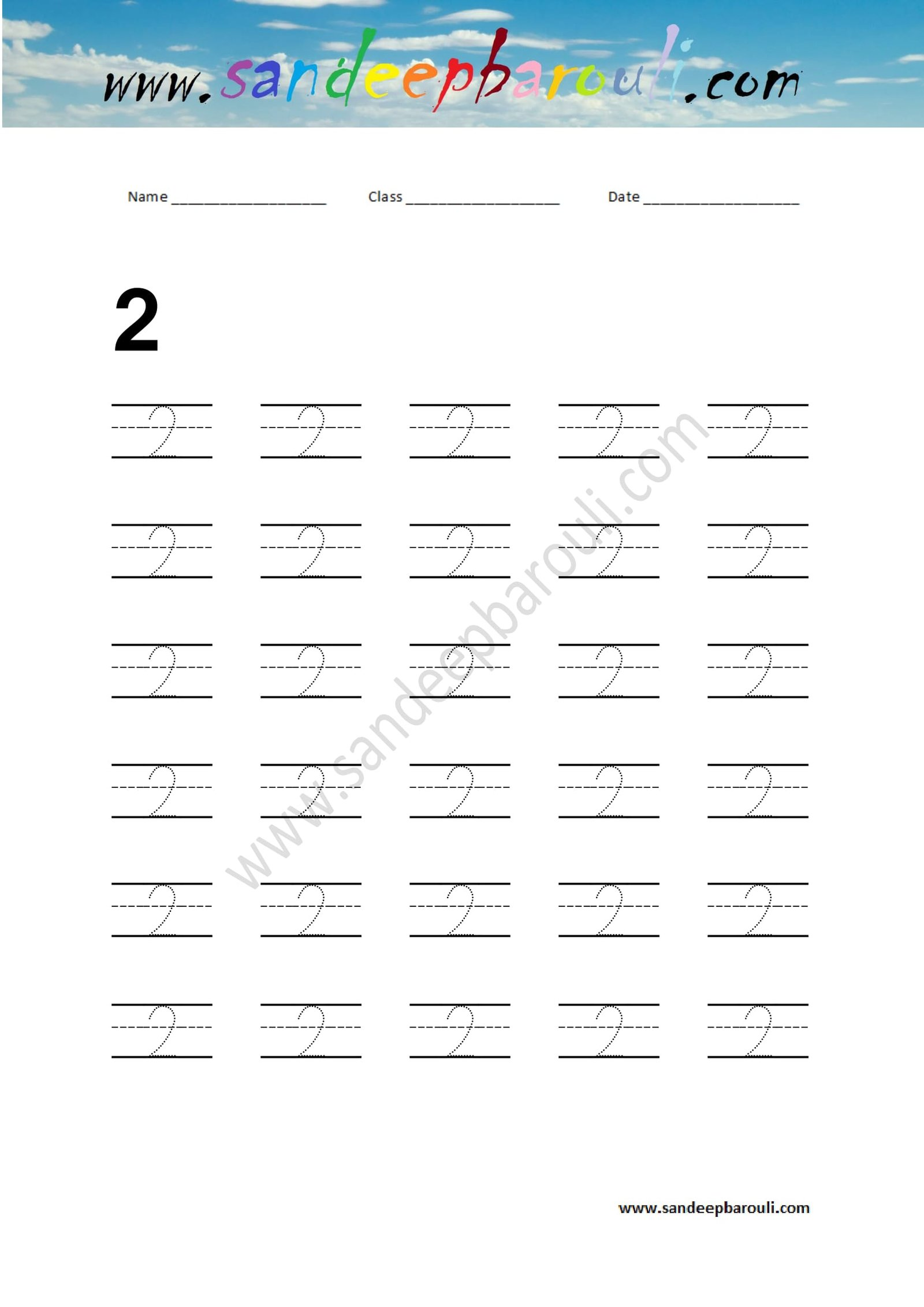# Number Writing Practice – (Number 2)

Math Worksheets for Kids 9

Number Writing Practice – (Number 2)

How to take printout of this worksheet?

Follow these steps:

Step 1. Click on worksheet.

Step 2. Right click on mouse.

Step 3. Save your worksheet as JPEG format.

Step 4. Take printout of JPEG file.

Thank You !!Writing Practice Number 0

Writing Practice Number 1

Writing Practice Number 2

Writing Practice Number 3

Writing Practice Number 4

Writing Practice Number 5

Writing Practice Number 6

Writing Practice Number 7

Writing Practice Number 8

Writing Practice Number 9

Writing Practice Number 10

Writing Practice Number 20

Writing Practice Number 30

Writing Practice Number 40

Writing Practice Number 50

Writing Practice Number 60

Writing Practice Number 70

Writing Practice Number 80

Writing Practice Number 90

##### Home Math Worksheets

Download as a PDF